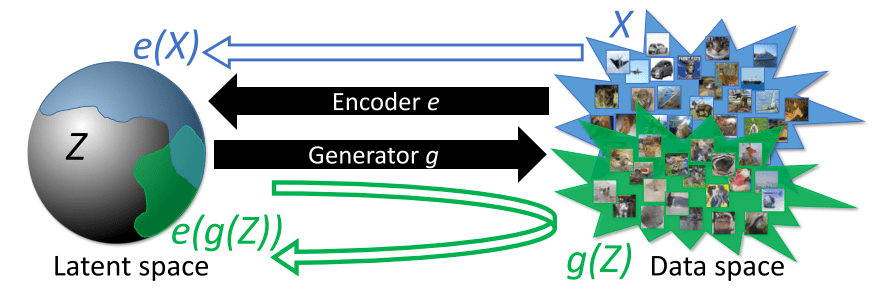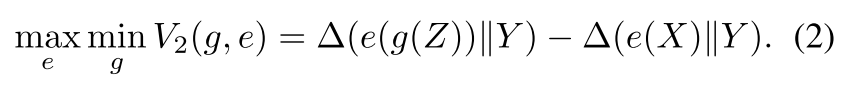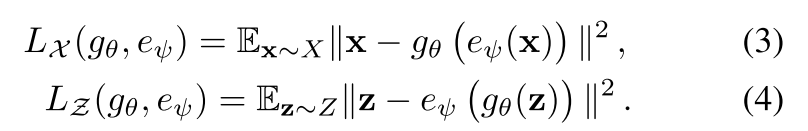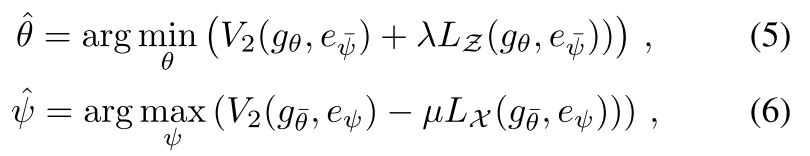New autoencoder-like generative network, called Adversarial Generator-Encoder Networks (AGE Network), does not have any discriminators, which makes the entire architecture much simpler than some recently-proposed GANs, but with nearly the same-level performance

## 1. Introduction

This paper proposes a new autoencoder-like generative network, called Adversarial Generator-Encoder Networks (AGE Network). This model is special because this AGE does not have any discriminators, which makes the entire architecture much simpler than some recently-proposed GANs, but with nearly the same-level performance of sample generation.

## 2. MethodsAs the figure above shows, this AGE network only has two components: one encoder (e) and one generator (g). The generator maps latent vectors into sample space, while the encoder maps both real samples and fake samples into latent space.

Normally, generative adversarial networks (GANs) only have one generator and one discriminator. The adversarial game is right between them: The generator is trained to map the random latent vector into sample space to obtain fake samples, which are as similar to real ones as possible; the discriminator, often a binary classifier, is trained to distinguish the fake samples from real samples.

But in this project, the authors set up the adversarial game as follows: The generator is required to generate indistinguishable samples, but at the same time, the encoder is to construct a latent space to separate the real samples from fake samples adversarially.

The corresponding objective function is defines in equation 2:where the operator “Delta()” measures the divergence between two distributions. The trick here is that the authors don’t compare the distributions of e(g(Z)) and e(X) directly. Instead, they use a canonical distribution Y as a reference, and compare “Delta 1” (the difference between e(g(Z)) and Y) with “Delta 2” (the difference between e(X) and Y). This way, by picking a suitable Y, the divergence evaluations are more stable than a direct comparison of “Delta(e(g(Z)) || e(X))”, because distributions of “e(g(Z))” and “e(X)” are defined implicitly and it is only possible to sample from them.

For the sample-level reciprocity, the authors introduce two loss functions as equation 3 and 4:These two loss functions are common L2 loss functions. L_x computes L2 loss in samples space, similar to a normal autoencoder. L_z is the L2 loss in latent vector space. With the functions above, the final objective functions for the generator and encoder are defined as equation 5 and 6:where theta_hat and phi_hat represent the value of the encoder and generator parameters at the moment of optimization. The terms with lambda and mu are Lagrange-Multipliers to constrain the optimization problem.

## 3. Experiments and Results

For the divergence measurement between a distribution Q and a distribution Z (here the uniform distribution on M-dimensional sphere is S^M), the authors defines equation 7 as follows:The generator and encoder here have a similar structure to the generator and discriminator in DCGAN. But their encoder is required to output a vector rather than a single number. They expand the last layer of the discriminator to M dimensions and replace the sigmoid at the end with a normalization layer.

The authors compare their generation results with DCGAN as shown in the following figure:Sub-figure (c) is the generated images without reconstruction loss, and sub-figure (d) is the generated image with the full objective functions. This model turns out to visually resemble DCGAN in terms of quality and diversity. The following is a further comparison between AGE, ALI and VAE:In this experiment, the generator is never supervised by the reconstruction loss of equation (3), which is used only for the encoder updates. Figure 9 shows that loss 3 leads to blurry result as normal autoencoder:## 4. Conclusion

This paper introduces a novel approach for simultaneous learning of generation and inference networks from unlabeled data. The objective of the game considers divergences between distributions rather than discrimination at the level of individual samples.

## 5. Thoughts from the Reviewer

This paper gives us a new way to design generative adversarial networks: Measure the divergence between the real and fake sample-distributions instead of classifying them. This new method can avoid some known pitfalls to a certain extent, such as mode collapse in prior GANs . This paper also shows their objective function is able to learn generators that produces high-quality samples even without reconstruction loss.

The problem of this method could be that the details of individual sample are not well enough generated. Because they measure the divergence of the distribution rather than discriminate samples, the details (edges or some semantic parts) are not considered.

One possible improvement could be cascading one more semantic parsing network after this AGE network, so a semantic loss could be added to the objective function to regularize the generated images, and thus both the entire distribution and individual samples could be better generated.

The authors published their codes on github: https://github.com/DmitryUlyanov/AGE

Paper Source: https://arxiv.org/abs/1704.02304

Author: Yiwen Liao | Technical Reviewer: Joshua Chou